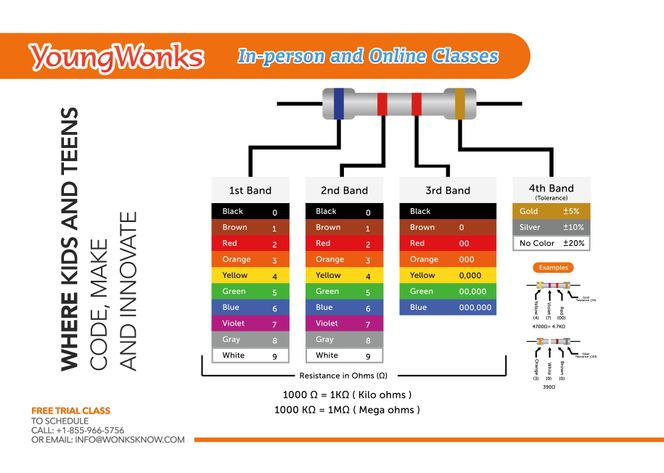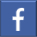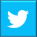# Finding the resistance of a resistor

## Where we tell you how to use the color-coding technique to find the resistance of a resistorSep 14, 2018    By Team YoungWonks *

Resistor color code is commonly used to find the resistance of a resistor. The color coding technique is explained in this blog.

But before that let’s take a quick look at what is a resistor. A resistor is a passive two-terminal electrical component that implements electrical resistance as a circuit element. In electronic circuits, resistors are used to reduce current flow, among other uses.

All resistors wear a certain number of colored bands; in this blog, we will be looking at how one can find the resistance of four-band resistors using the color-coding method.

The color coding technique is quite simple and involves each color being associated with a number between 0 and 9.

• First, we need to identify which of the four bands represents the first band. In four-band resistors, always start by looking for the gold or silver colored band. This gold/ silver band is the tolerance band as it indicates the range by which the resistor’s resistance value can differ from its claimed value. For example, a gold colored band indicates that the resistance of the resistor might be +/- 5% from the claimed value; i.e. it could be 5 % greater or 5% lower than the claimed value.

• The band at the other end of the resistor represents the first band. To calculate the resistance of the resistor, write the numbers associated with the first and second colored bands right next to each other and in the respective order. This will result in a two digit number. Now look at the third color and check the number associated with it. Write the same number of zeros on the right of the two digit number you have. Together, the resulting number shall give you the resistance of the resistor.

In the above image, we see a resistor where the first band is blue, the second and third bands are red and the fourth band is gold. Now, the first color blue is associated with 6 and the second color red is associated with the number 2. Placing these two numbers (6 and 2) next to each other in the corresponding order will give you the two digit number 62. To this, we add two zeros on the right as the third color red is associated with the number 2. This gives us a resistor value of 6200 ohms. You can also write it as 6.2 kiloohms or 6.2 KΩ.

This is how one can use the color coding technique to find the resistance of a resistor.

Shared below is a video explaining the same:*Contributors: Written by Vidya Prabhu; Image by Abhishek Aggarwal; Video post production by Leonel Cruz

This blog is presented to you by YoungWonks. The leading coding program for kids and teens.

YoungWonks offers instructor led one-on-one online classes and in-person classes with 4:1 student teacher ratio.

Sign up for a free trial class by filling out the form below:

By clicking the "Submit" button above, you agree to the privacy policyShare on FacebookShare on Twitter
help